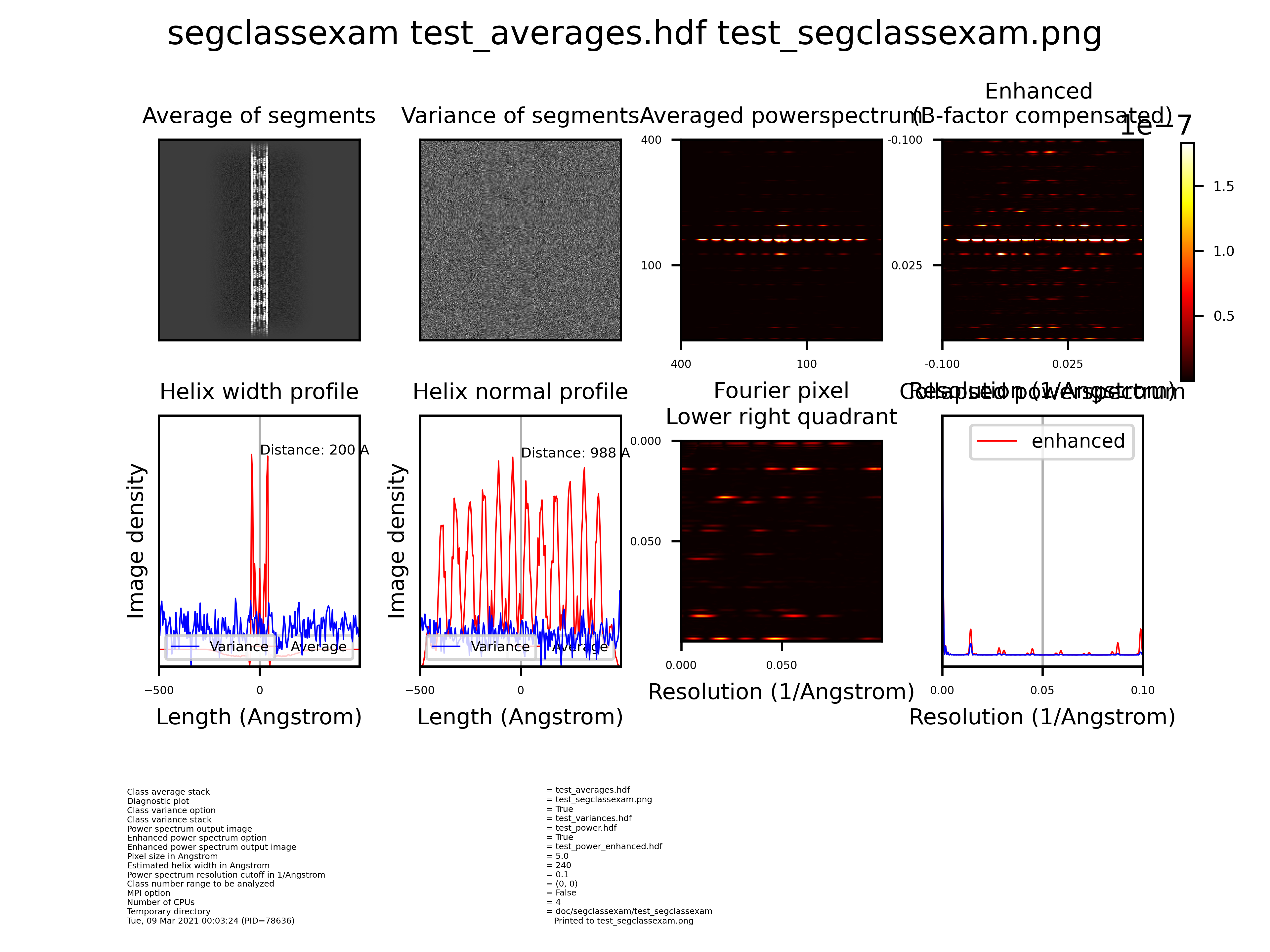# Segclassexam¶

Program to examine helix classes to compute their collapsed (1D) and 2D power spectrum and width profile of helicesInput: Class average stack Output: Diagnostic plot

## Parameters¶

Parameter

Example (default)

Description

Class average stack

avgerages.hdf

Class average stack: accepted file formats (hdf).

Diagnostic plot

segclassexam_diag.pdf

Output diagnostic plot: accepted file formats (pdf, .png, .bmp, .emf, .eps, .gif, .jpeg, .jpg, .ps, .raw, .rgba, .svg, .svgz, .tif, .tiff).

Power spectrum output image

power.hdf

Output power spectrum image: accepted file formats (hdf).

Pixel size in Angstrom

1.163

Pixel size is an imaging parameter (accepted values min=0.001, max=100).

Estimated helix width in Angstrom

200

Generous width measure of helix required for rectangular mask (accepted values min=0, max=1500).

Class number range to be analyzed

(3, 5)

Class number range to be analyzed (1st class is 0) (accepted values min=0, max=2000).

## Sample parameter file¶

You may run the program in the command line by providing the parameters via a text file:

```segclassexam --f parameterfile.txt
```

Where the format of the parameters is:

```Class average stack                      = avgerages.hdf
Diagnostic plot                          = segclassexam_diag.pdf
Power spectrum output image              = power.hdf
Pixel size in Angstrom                   = 1.163
Estimated helix width in Angstrom        = 200
Class number range to be analyzed        = (3, 5)
```

Parameter

Example (default)

Description

Class variance option

False

Check if variances of classes are to be analyzed.

Class variance stack

variances.hdf

Stack: accepted image file formats (spi, hdf, img, hed)

MPI option

True

OpenMPI installed (mpirun).

Number of CPUs

8

Number of processors to be used (accepted values min=1, max=1000).

Temporary directory

/tmp

Temporary directory should have fast read and write access.

## Sample parameter file (intermediate level)¶

You may run the program in the command line by providing the parameters via a text file:

```segclassexam --f parameterfile.txt
```

Where the format of the parameters is:

```Class average stack                      = avgerages.hdf
Diagnostic plot                          = segclassexam_diag.pdf
Class variance option                    = False
Class variance stack                     = variances.hdf
Power spectrum output image              = power.hdf
Pixel size in Angstrom                   = 1.163
Estimated helix width in Angstrom        = 200
Class number range to be analyzed        = (3, 5)
MPI option                               = True
Number of CPUs                           = 8
Temporary directory                      = /tmp
```

Parameter

Example (default)

Description

Enhanced power spectrum option

True

Option to enhance power spectrum to compensate for decay of amplitudes.

Enhanced power spectrum output image

power_enhanced.hdf

Output enhanced power spectrum image (compensated for decay of amplitudes): accepted file formats (hdf).

Power spectrum resolution cutoff in 1/Angstrom

0.15

Images are binned to obtain a suitable power spectrum (accepted values min=0, max=1).

## Sample parameter file (expert level)¶

You may run the program in the command line by providing the parameters via a text file:

```segclassexam --f parameterfile.txt
```

Where the format of the parameters is:

```Class average stack                      = avgerages.hdf
Diagnostic plot                          = segclassexam_diag.pdf
Class variance option                    = False
Class variance stack                     = variances.hdf
Power spectrum output image              = power.hdf
Enhanced power spectrum option           = True
Enhanced power spectrum output image     = power_enhanced.hdf
Pixel size in Angstrom                   = 1.163
Estimated helix width in Angstrom        = 200
Power spectrum resolution cutoff in 1/Angstrom = 0.15
Class number range to be analyzed        = (3, 5)
MPI option                               = True
Number of CPUs                           = 8
Temporary directory                      = /tmp
```

## Command line options¶

When invoking segclassexam, you may specify any of these options:

```usage: segclassexam [-h] [--g] [--p] [--f FILENAME] [--c] [--l LOGFILENAME] [--d DIRECTORY_NAME] [--version] [--class_variance_option]
[--enhanced_power_spectrum_option] [--mpi_option]
[input_output [input_output ...]]

Program to examine helix classes to compute their collapsed (1D) and 2D power spectrum and width profile of helices

positional arguments:
input_output          Input and output files

optional arguments:
-h, --help            show this help message and exit
--g, --GUI            GUI option: read input parameters from GUI
--p, --promptuser     Prompt user option: read input parameters from prompt
--f FILENAME, --parameterfile FILENAME
File option: read input parameters from FILENAME
--c, --cmd            Command line parameter option: read only boolean input parameters from command line and all other parameters will be assigned
from other sources
--l LOGFILENAME, --logfile LOGFILENAME
Output logfile name as specified
--d DIRECTORY_NAME, --directory DIRECTORY_NAME
Output directory name as specified
--version             show program's version number and exit
--class_variance_option, --cla
Check if variances of classes are to be analyzed. (default: False)
--enhanced_power_spectrum_option, --enh
Option to enhance power spectrum to compensate for decay of amplitudes. (default: False)
--mpi_option, --mpi   OpenMPI installed (mpirun). (default: False)
```